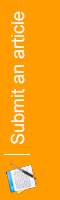#### Berhulov A. S.

ВУЗ / Место работы:

Kyiv, Ukraine

Number of publications: 2

Summary: Subject of interstellar flights remains actual taking into account limitedness of the terrestrial resources, overpopulation of the planet, and demographic, sociopolitical and ecological problems at the planet. A constructive solution is submitted of the construction of a spacecraft moving with acceleration resting upon electromagnetic fields of the Solar system and the Galaxy. Calculation of the current intensity within the circuit and charge value at the object's useful surface necessary for the achievement of acceleration equal to at arbitrary direction in the space is conducted.

Keywords: electromagnetic fields of the Galaxy; magnetic levitation; spacecraft; Lorentz force; dependence of mass on velocity; redistribution of charges by surface.

Branch of science: Technical sciences

Summary: There is a numerical and analytical approach to research of stress-strain state of an anisotropic composite finite element field with different boundary conditions on the surface. The problem is solved using a three-dimensional model of the theory of elasticity. The system of differential equations is reduced to one-dimensional problem through the use of spline-collocation in the two coordinate directions. Boundary value problem for a system of ordinary differential equations of higher order is decided with the stable numerical method of discrete orthogonalization.

Key words: stress-strain state, partial differential equations, method of spline collocation, method of discrete orthogonalization, anisotropic finite element region.

Branch of science: Physics and mathematics